Volume 14, Issue 4 (July & August 2023)                   BCN 2023, 14(4): 519-528 | Back to browse issues page

BibTeX | RIS | EndNote | Medlars | ProCite | Reference Manager | RefWorks
Send citation to:Mehdizadehfar V, Ghassemi F, Fallah A. Brain Connectivity Estimation Pitfall in Multiple Trials of Electroencephalography Data. BCN 2023; 14 (4) :519-528
URL: http://bcn.iums.ac.ir/article-1-2215-en.html
1- Department of Bioelectric, School of Biomedical Engineering, Amirkabir University of Technology, Tehran, Iran.
Full-Text [PDF 1565 kb]       |   Abstract (HTML)
Full-Text:
1. Introduction
All brain functions, including sensation, perception, cognition, and any human action, are performed by dynamically complex neuronal networks in the brain (Omidvarnia et al., 2014) by orthogonalization of the strictly causal multivariate autoregressive model coefficients, to minimize the effect of mutual sources. The novel measure, generalized orthogonalized partial directed coherence (gOPDC). The study of these networks helps to better understand the mechanisms of brain neurodevelopment and its related disorders. There is a growing interest in studying the brain to identify connectivity and interactions among distributed brain regions (Bastos & Schoffelen, 2016). Several imaging techniques can be utilized to evaluate brain connectivity, such as functional magnetic resonance imaging (fMRI), magnetoencephalography (MEG), and electroencephalography (EEG) (Cociu et al., 2017) fMRI and diffusion tensor imaging (DTI) have traditionally been used to find biomarkers for autism, but there have been very few attempts for a combined or multimodal approach of EEG, fMRI and DTI to understand the neurobiological basis of autism spectrum disorder (ASD). EEG is often an appropriate technique for studying brain connectivity due to its high temporal resolution, non-invasive nature, and low cost (Williams et al., 2018). Two critical explanations of brain connectivity have been suggested, functional and effective connectivity (non-directed and directed connectivity, respectively) (Astolfi et al., 2007) its modification known as direct DTF (dDTF). Functional connectivity is inferred based on temporal correlations between measurements of neuronal activity. In contrast, effective connectivity refers to the model of causal effects that describes the influence of a neural unit on others (Friston, 2011). Therefore, effective connectivity demonstrates the direction of information flow in the brain.
Once the EEG signals have been recorded, the signal-processing specialist is confronted with the challenge of quantifying the neural interactions and providing a reliable interpretation of the findings (Wang et al., 2014). When the number of trials/observations used to compute connectivity metrics is different, in other words, different length of data is involved in the calculation of connectivity metrics, estimated connectivity, and consequently its neurophysiological interpretations vary across different trial counts. Connectivity metrics are often affected by the length of the data. A reason for this fact is that many connectivity measures are based on the magnitude of a quantity, which always has a positive value, therefore the difference of measures computed with different data lengths will never be zero. A set of human emotional faces consists of happy, sad, and anger, and a set of human emotional faces consists of happy, sad, and anger zero. The effect of this fact often depends on the data points of data, the smaller the data points, the larger the effect (Bastos & Schoffelen, 2016). For some measures, such as coherence, analytic estimates of this effect can be estimated and applied, but cannot be generalized to all metrics (Bokil et al., 2007)”mendeley”: {“formatted citation”: “(Bokil et al., 2007). Another suggestion to address this problem is to utilize the inferential statistical framework, based on a non-parametric/parametric statistical test, where GC estimates cannot be generalizable to 5, 10, 100, 500, and 1000 trials. The vertical axis represents the average GC metric±1 standard deviation across 100 realizations (simulated data). This paper was conducted to clarify the confusion in connectivity analysis when using multiple trials of EEG data. The analysis pitfall that a researcher may encounter in performing connectivity analysis on real data is investigated using the simulated data and experimental data.

2. Materials and Methods
The flowchart illustrated in Figure 1 outlines the summary of the procedure in the current study. The presented procedures are evaluated using the simulated data and experimental data. The flowchart summarizes the essential steps. As shown in Figure 1A1, two synthetic signals are generated. Experimental EEG data are prepro-cessed according to Figure 1A. The multivariate autoregressive (MVAR) model representation of signals is utilized to find out the information flow between pairs of signals and directional interactions between them as shown in Figure 1B. Finally, the connectivity measure is extracted and compared in different trial numbers which are summarized in Figure 1C. All of these steps are described in detail below.Multivariate autoregressive (MVAR) model
Directed connectivity measures can be quantified with various methods, which are divided into two groups of data-driven and model-based methods (reviewed in (Lehnertz, 2011)). Among these, MVAR has been widely utilized for biological signal analysis (Faes et al., 2012) partial coherence. The MVAR process is a time-frequency domain statistical tool to model directional and causal information flow between EEG electrodes. In these model-based procedures, a parametric MVAR model fits observed data time series using some techniques, such as multivariate lattice algorithms, state-space models or least‐squares methods (Mullen, 2010). In the last decade, an increasing number of model-based connectivity measures, closely related to Granger’s definition of causality, have been proposed. GC-based connectivity measures were considered in the current paper.
In 1969, Granger expressed a comprehensive formalization of GC (Granger & Aug, 1969). GC states that if one stochastic process X2(t) contains information in past values that permits a more accurate prediction of X1(t), then X2(t) could be called a casual to X1(t) (Blinowska, 2011). Assume X1(t) is represented by an AR model using P previous values of X1(t) with a prediction error e11 and A as the coefficient matrix (Equation 1).Then suppose X1(t) is represented using P previous values of X1(t) and P previous values of X2(t) with a prediction error e12 (Equation 2).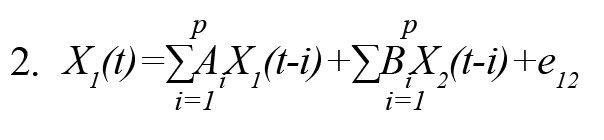If the variance of e11(δ211(e11)) is more than the variance of e12(δ212(e12)), then it is an explanation of a causal interaction from X2(t) to X1(t).

Segmentation-based MVAR model
The concept of segmentation-based MVAR is similar to windowing techniques. The sliding window of length W from the data is extracted and the MVAR model is fitted to this data. By moving the sliding window and repeating the procedure, the MVAR coefficient matrix is obtained. Sufficient data points to fit the model is a crucial issue accurately. Let X(t)=x1,...xT is an M-dimensional time-series EEG data of length T (M: Electrodes of EEG data, T: Time points per electrode). In the general case, we have M2P coefficients to estimate (M: The number of EEG electrodes, P: The order of model), which requires a minimum of M2P data samples. It is required to have much more than these data samples. When multiple trials are available, each trial can be assumed a random sample from the same stochastic process and averaging of trials reduces the model bias. Therefore, for accurate MVAR estimation, the data from all trials are used. After obtaining the frequency‐domain representation of the model, several quantities can be computed related to the information flow, oscillations, and coupling in neuronal systems based on model parameters for each trial separately. In the connectivity measure of multiple trials, an essential stage is to take a vector summation operation followed by a total number of trials-normalization stage. Compared to calculated connectivity measures from samples with different numbers of trials, it was observed that an overestimation of connectivity may occur in the condition with the smallest number of samples (low number of trials) (Friston, 2011). Therefore the larger trial numbers result in better model parameter estimation.

Granger causality (GC)
GC and Granger-based measures are susceptible to quantify bi-directional interactions. Originally, the concept of GC was defined in the time domain, then the extension to the frequency domain was formulated by Geweke (Geweke, 1982). Two quantities are required to computee frequency domain-GC, the covariance of the AR-model’s residuals (∑), and the spectral transfer matrix H(ω) (defined as A-1[ω]).
The following fundamental identity holds: H(ω)∑H(ω)*=S(ω) (Wilson, 1972), with S(ω) being the cross-spectral density matrix for X1 and X2 at frequency ω. From the residuals’ covariance matrix, the spectral transfer matrix, and the cross-spectrum, the GC in the frequency domain can be computed as follows (Equation 3):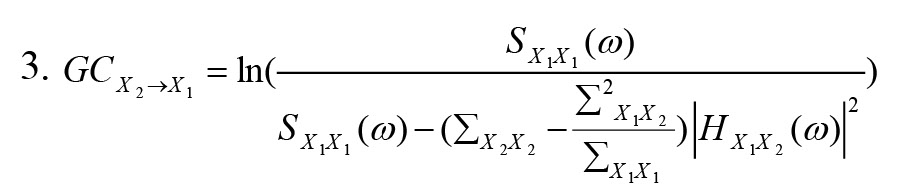Partial directed coherence (PDC)
The PDC was defined in the following form (Baccalá & Sameshima, 2001) (Equation 4):In this equation, Aij(f) is the Fourier transform of MVAR model coefficients A(t) and aj(f) is a column with index j of A(f) matrix.

3. Results
Pipeline validation

To simulate the effect of trial number on connectivity calculation, two simulated data were generated with known AR coefficients, a sampling rate of 400 Hz, and 500 ms trial length. After extracting the GC, based on 5, 10, 100, 500, and 1000 trials, simple auto-regressive models were calculated for each condition. After selecting the model parameters, the model order was tested between 1 and 20 to select the best order using AIC information criteria. The proposed model was validated using three criteria of whiteness, consistency, and stability. Finally, a model that met all three validation criteria is selected.
Two popular connectivity measures, GC and PDC, are selected to investigate in the present paper. Here, 100 simulations of a simple AR model were realized and the average and the standard deviation across realizations are considered. The simulations showed that the estimating GC corresponding to small trial numbers resulted in an average value of connectivity that is significantly higher and also more variable over different estimates compared to conditions with higher trial numbers. By increasing the number of trials, the AR model is more appropriately fitted to the data and hence the GC results are more reliable. Figure 2 shows the results of this simulation. A similar result is obtained for the PDC metric. As shown in Figure 3, the average PDC value decreases with increasing the number of trials and also its variability decreases. The obtained results of these two simulations quite agree.Figure 4 and Figure 5 show the value of GC and PDC connectivity metrics throughout the frequency band for different trial numbers respectively. As shown in Figure 4 and Figure 5, the values of connectivity are lower for higher trial numbers.Experimental data
A set of human emotional faces, including happy, sad, and anger was selected from the extended Cohn-Kanade (ck+) dataset (Lucey et al., 2010). The facial expressions were presented on a computer screen for a duration of 3000 ms and immediately replaced by a black screen for 1000 ms. The task was developed using Psychtoolbox software, version 3.0.14 (Brainard et al., 1997). Participants were asked to determine the emotion by pressing a key using a gamepad with three buttons corresponding to each expression. They were requested to look at the center of the screen and attempt to reduce blinks during the data acquisition. EEG signal was continuously recorded using active electrodes situated on a standard cap according to the 10-20 system using a g.tec amplifier and digitized at 1200 Hz. Impedances of all active electrodes were kept below 10kΩ throughout data acquisition (Mehdizadehfar et al., 2020).
The proposed pipeline was applied to real EEG data. Data were analyzed for two electrodes in frontal (F4) and occipital (O2) regions. After standard preprocessing, including band-pass filtering (0.5-60 Hz), removing ocular artifact, epoch (pre-stimulus time=500 ms, post-stimulus time=1500 ms), and removing bad epochs, GC and PDC were extracted for 5, 10, 20, and 60 trials. Estimated GC corresponding to small trial numbers resulted in an average GC value that was higher over different estimates compared to conditions with higher trial numbers (Figure 6).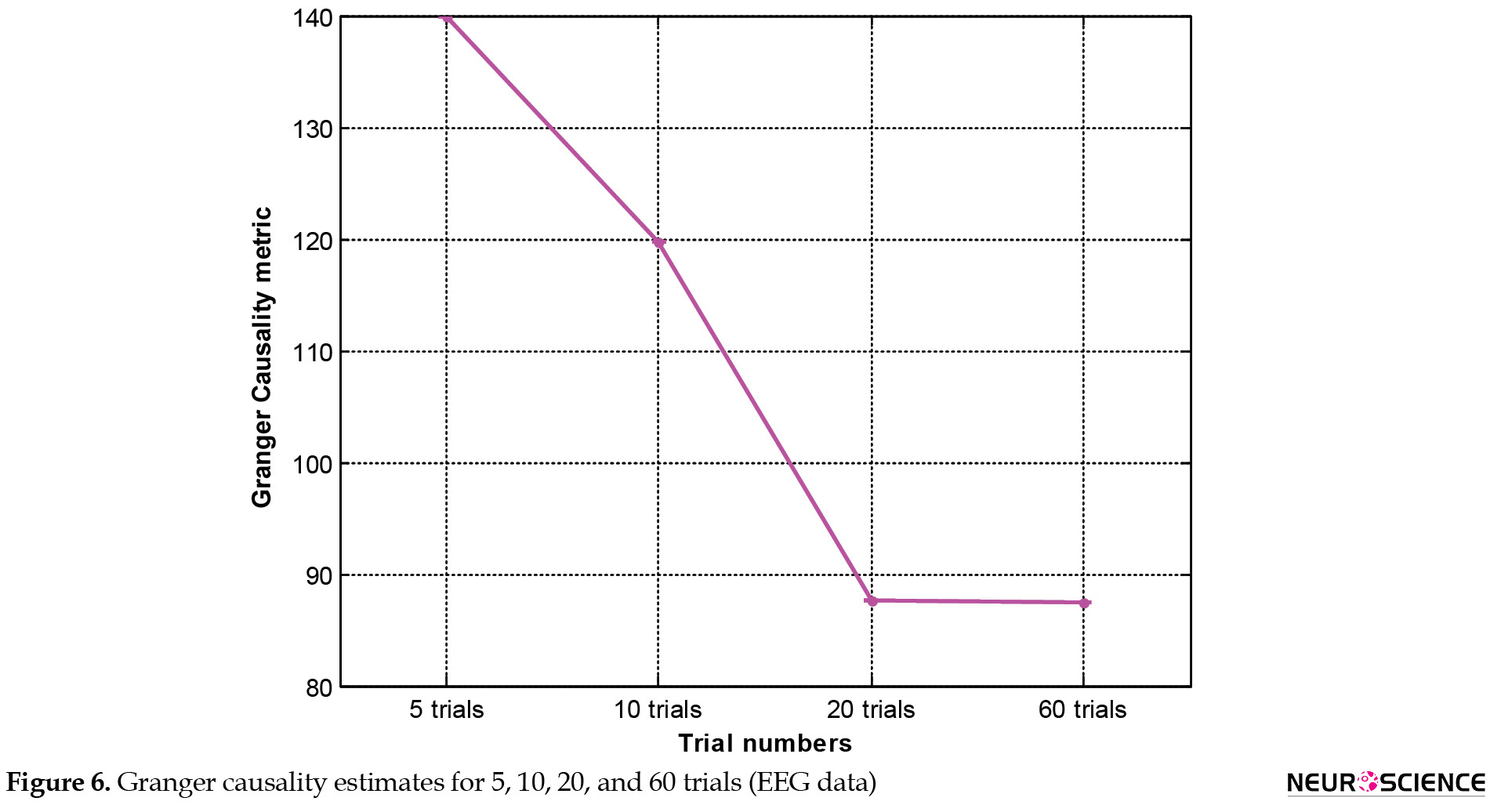As shown in Figure 7, the value of average PDC decreases with increasing the number of trials.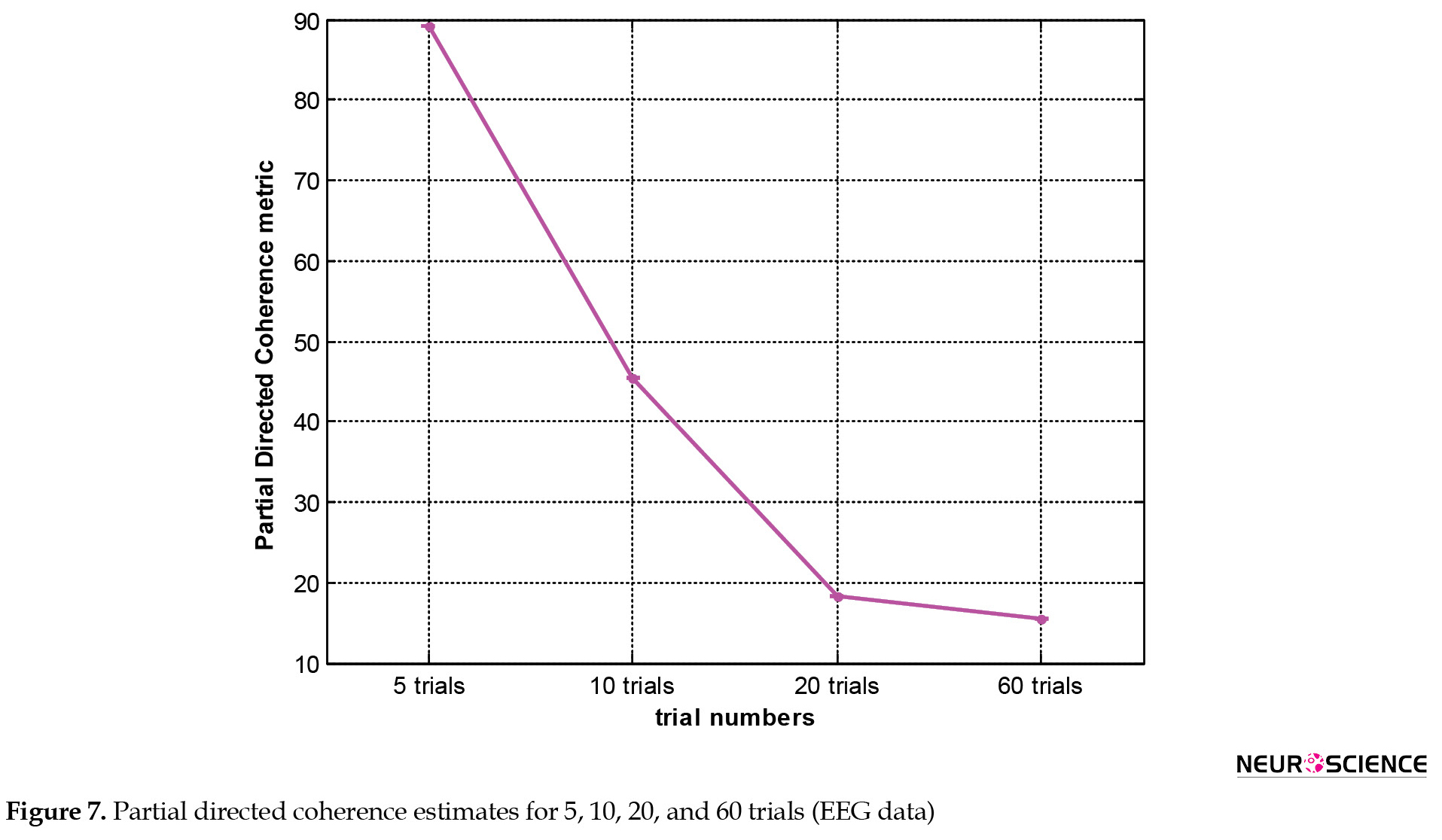The obtained results agree well with the findings of simulated data and the decreasing trend in the connectivity measure has the same trend in simulated data and real EEG data. Figure 8 and Figure 9 show the value of GC and PDC connectivity between two EEG electrodes (F4 and O2) throughout the frequency band for different trial numbers, respectively. As shown in Figure 8 and Figure 9, the values of connectivity are lower for higher trial numbers.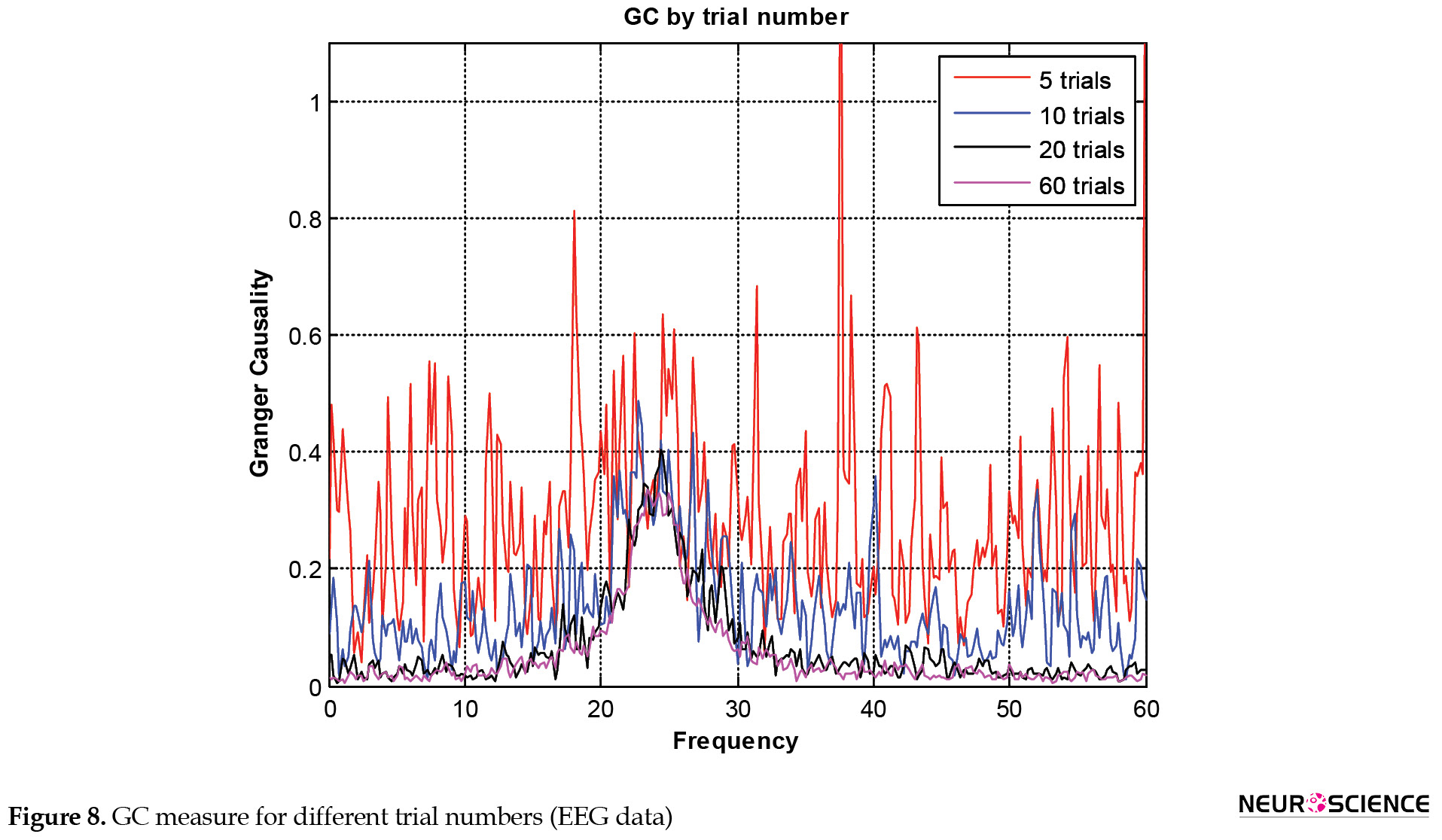4. Discussion
This paper was conducted to study brain connectivity estimation pitfalls related to multiple trials of EEG data. When attempting to compute connectivity from data with many observation epochs, the question arises whether brain connectivity is calculated for each trial and then average or for the averaged trials. When the connectivity measures were calculated on trials, it must be interpreted as a measure of dependency between averaged trails responses, rather than the underlying neural processes. Since this can be difficult to interpret, it is suggested to fit connectivity models to the ensembles of trials instead of describing information transfer. Here, the number of trials used to calculate connectivity should be considered. Estimating connectivity corresponding to small trial numbers (5 and 10 trials in our simulations) resulted in a higher average value. For a larger number of trials (100, 500, and 1000 trials in our simulations), results are more reliable. Therefore, the larger the trial number, the better the model parameter estimation. The analysis of connectivity metrics for different lengths of EEG data also confirmed the results of simulation data. By increasing the trial numbers, the values of connectivity measures are decreased and converged.

5. Conclusion
This paper presented a connectivity estimation pitfall when using multiple trials of EEG data. GC and PDC connectivity estimation methods, as a frequency domain demonstration of the directed relationship between pairs of signals, were applied to simulated synthetic time series. A significant decrease in the value of connectivity measures and their variability was observed by increasing the number of trials, thus confirming the overestimation of connectivity in small trial numbers. Therefore, for the larger trial numbers, connectivity estimation will be more accurate and therefore result in a reliable interpretation of connectivity networks in the brain. The pitfall investigated in this paper is a general issue and it is independent of the type of task used in data recording and the method of connectivity measurement. It is hoped that this clarification will be effective in reducing the confusion of researchers and results in a proper inference.

Ethical Considerations
Compliance with ethical guidelines

All ethical principles are considered in this article. The participants were informed of the purpose of the research and its implementation stages. They were also assured about the confidentiality of their information and were free to leave the study whenever they wished, and if desired, the research results would be available to them. A written consent has been obtained from the subjects. Principles of the Helsinki Convention was also observed.

Funding
The paper was extracted from the PhD thesis of Vida Mehdizadehfar, approved by the Department of Bioelectric, Amirkabir University of Technology.

Authors' contributions
Conceiving and study design: Vida Mehdizadehfar, and Farnaz Ghassemi; Conducting the analysis: Vida Mehdizadehfar; Interpreting the findings: All authors; Writing the manuscript: Vida Mehdizadehfar; Review and editing: Farnaz Ghassemi and Ali Fallah.

Conflict of interest
The authors declared no conflict of interest.

Acknowledgments
The authors would like to thank the National Brain Mapping Lab (NBML) for their contributions to data collection.

References
Astolfi, L., Cincotti, F., Mattia, D., Marciani, M. G., Baccala, L. A., & de Vico Fallani, F., et al. (2007). Comparison of different cortical connectivity estimators for high-resolution EEG recordings. Human Brain Mapping, 28(2), 143–157. [DOI:10.1002/hbm.20263] [PMID]
Baccalá, L. A., & Sameshima, K. (2001). Partial directed coherence: A new concept in neural structure determination. Biological Cybernetics, 84(6), 463–474. [DOI:10.1007/PL00007990] [PMID]
Bastos, A. M., & Schoffelen, J. M. (2016). A tutorial review of functional connectivity analysis methods and their interpretational pitfalls. Frontiers in Systems Neuroscience, 9, 175. [DOI:10.3389/fnsys.2015.00175] [PMID]
Blinowska K. J. (2011). Review of the methods of determination of directed connectivity from multichannel data. Medical & Biological Engineering & Computing, 49(5), 521–529.[DOI:10.1007/s11517-011-0739-x] [PMID]
Bokil, H., Purpura, K., Schoffelen, J. M., Thomson, D., & Mitra, P. (2007). Comparing spectra and coherences for groups of unequal size. Journal of Neuroscience Methods, 159(2), 337–345.[DOI:10.1016/j.jneumeth.2006.07.011] [PMID]
Brainard D. H. (1997). The psychophysics toolbox. Spatial Vision, 10(4), 433–436. [DOI:10.1163/156856897X00357] [PMID]
Cociu, B. A., Das, S., Billeci, L., Jamal, W., Maharatna, K., & Calderoni, S., et al. (2017). Multimodal functional and structural brain connectivity analysis in autism: A preliminary integrated approach with EEG, fMRI and DTI. IEEE Transactions on Cognitive and Developmental Systems, 10(2), 213-226. [DOI:10.1109/TCDS.2017.2680408]
Faes, L., Erla, S., & Nollo, G. (2012). Measuring connectivity in linear multivariate processes: definitions, interpretation, and practical analysis. Computational and Mathematical Methods in Medicine, 2012, 140513. [DOI:10.1155/2012/140513] [PMID]
Friston K. J. (2011). Functional and effective connectivity: A review. Brain Connectivity, 1(1), 13–36. [DOI:10.1089/brain.2011.0008] [PMID]
Geweke, J. (1982). Measurement of linear dependence and feedback between multiple time series. Journal of the American Statistical Association, 77(378), 323-324. [DOI:10.1080/01621459.1982.10477803]
Granger, C. W. J., & Aug, N. (1969). Investigating causal relations by econometric models and cross-spectral methods. Econometrica, 37(3), 424-438. [DOI:10.2307/1912791]
Lehnertz K. (2011). Assessing directed interactions from neurophysiological signals--an overview. Physiological Measurement, 32(11), 1715–1724. [DOI:10.1088/0967-3334/32/11/R01] [PMID]
Lucey, P., Cohn, J. F., Kanade, T., Saragih, J., Ambadar, Z., & Matthews, I. (2010). The extended cohn-kanade dataset (ck+): A complete dataset for action unit and emotion-specified expression. Paper presented at: 2010 IEEE Computer Society Conference on Computer Vision and Pattern Recognition - Workshops. San Francisco, USA, 13 June 2010. [DOI:10.1109/CVPRW.2010.5543262]
Mehdizadehfar, V., Ghassemi, F., Fallah, A., & Pouretemad, H. (2020). EEG study of facial emotion recognition in the fathers of autistic children. Biomedical Signal Processing and Control, 56, 101721. [DOI:10.1016/j.bspc.2019.101721]
Mullen, T. (2010). Source information flow toolbox ( SIFT ). San Diego: Swartz Center for Computational Neuroscience. [Link]
Omidvarnia, A., Azemi, G., Boashash, B., O'Toole, J. M., Colditz, P. B., & Vanhatalo, S. (2014). Measuring time-varying information flow in scalp EEG signals: Orthogonalized partial directed coherence. IEEE Transactions on Bio-Medical Engineering, 61(3), 680–693. [DOI:10.1109/TBME.2013.2286394] [PMID]
Wang, H. E., Bénar, C. G., Quilichini1, P. P., Friston, K. J., Jirsa, V. K., & Valdes-sosa, P. A., et al. (2014). A systematic framework for functional connectivity measures. Frontiers in Neuroscience, 8, 405. [DOI:10.3389/fnins.2014.00405]
Williams, N. J., Daly, I., & Nasuto, S. J. (2018). Markov model-based method to analyse time-varying networks in EEG Task-related data. Frontiers in Computational Neuroscience, 12, 76. [DOI:10.3389/fncom.2018.00076] [PMID]
Wilson, G. T. (1972). The factorization of matricial spectral densities. Applied Mathematics 23(4):420–426. [Link]
Type of Study: Original | Subject: Computational Neuroscience
Received: 2021/04/6 | Accepted: 2021/08/21 | Published: 2023/07/1Rights and permissionsThis work is licensed under a Creative Commons Attribution-NonCommercial 4.0 International License.